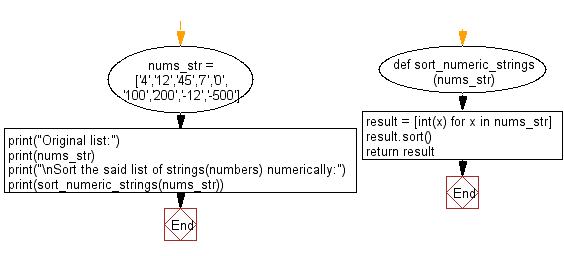﻿ Python: Sort a given list of strings(numbers) numerically - w3resource# Python: Sort a given list of strings(numbers) numerically

## Python List: Exercise - 139 with Solution

Write a Python program to sort a given list of strings(numbers) numerically.

Sample Solution:

Python Code:

``````def sort_numeric_strings(nums_str):
result = [int(x) for x in nums_str]
result.sort()
return result
nums_str = ['4','12','45','7','0','100','200','-12','-500']
print("Original list:")
print(nums_str)
print("\nSort the said list of strings(numbers) numerically:")
print(sort_numeric_strings(nums_str))
```
```

Sample Output:

```Original list:
['4', '12', '45', '7', '0', '100', '200', '-12', '-500']

Sort the said list of strings(numbers) numerically:
[-500, -12, 0, 4, 7, 12, 45, 100, 200]
```

Flowchart:## Visualize Python code execution:

The following tool visualize what the computer is doing step-by-step as it executes the said program:

Python Code Editor:

Have another way to solve this solution? Contribute your code (and comments) through Disqus.

What is the difficulty level of this exercise?

Test your Python skills with w3resource's quiz

﻿

## Python: Tips of the Day

Floor Division:

When we speak of division we normally mean (/) float division operator, this will give a precise result in float format with decimals.

For a rounded integer result there is (//) floor division operator in Python. Floor division will only give integer results that are round numbers.

```print(1000 // 300)
print(1000 / 300)```

Output:

```3
3.3333333333333335```